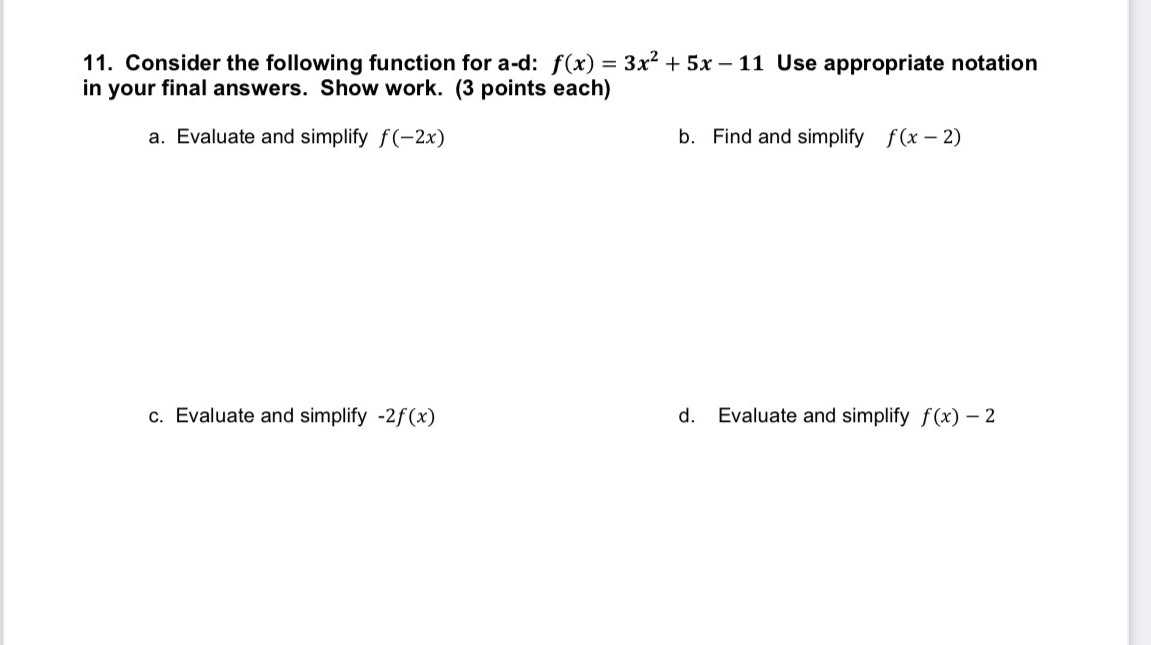### ¿Todavía tienes preguntas de matemáticas?

Pregunte a nuestros tutores expertos
Algebra
Pregunta11. Consider the following function for a-d: $$f ( x ) = 3 x ^ { 2 } + 5 x - 11$$ Use appropriate notation in your final answers. Show work. ($$3$$ points each)

a. Evaluate and simplify $$f ( - 2 x )$$

b. Find and simplify $$f ( x - 2 )$$

c. Evaluate and simplify $$- 2 f ( x )$$

d. Evaluate and simplify $$f ( x ) - 2$$

a)$$f(- 2x)= 12x^{2} - 10x- 11$$
b)$$f(x- 2)= 3x^2 - 7x- 9$$
c)$$- 2f(x)= -6x2 - 10x+ 22$$
d)$$f(x)- 2= 3x^2+ 5x- 13$$